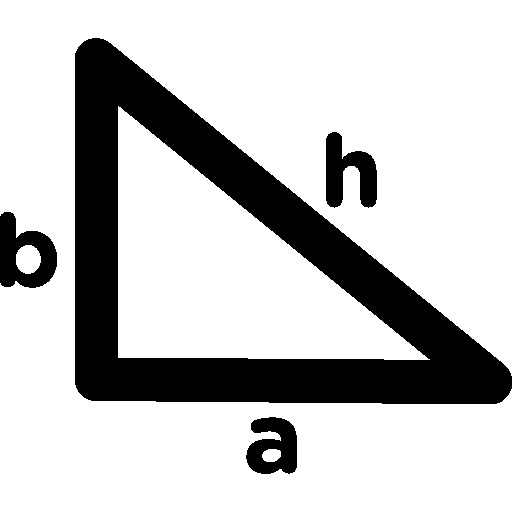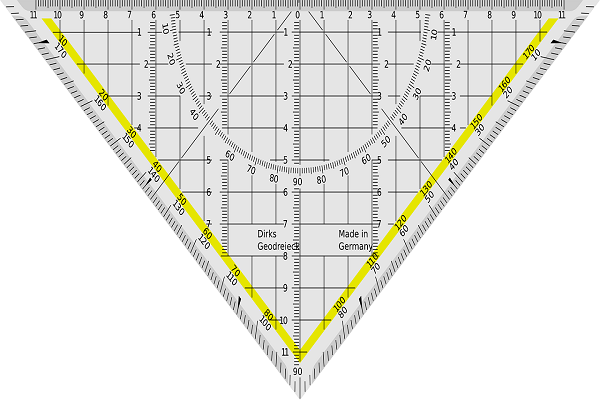# Everything You Need to Know About The Different Pythagoras Theorem Questions

Jul 28, 2021Have you heard of the Pythagoras theorem? Without any doubt, you must have. It is one of the most popular mathematical formulas. It shows the relationship between the different sides of the right-angled triangle and helps in the determination of the unknown side’s length. Moreover, it is known as one of the fundamental geometric concepts in mathematics.

When it comes to the Pythagoras theorem, there are a number of different types of questions that you may come across. Are you wondering how to solve them? Well, to make it easy for you, here are some questions and the ways to solve them effectively to get the right answers. Let’s explore!

### Determination of Whether a Given Triangle is a Right-angled Triangle or Not

How do you know whether a given triangle is a right-angled triangle or not? Well, it is quite simple and easy. However, before starting any kind of calculations, the first thing to do is to find out whether you have the lengths of the three sides of the triangles or not. Once you are sure that you have them all, you can go ahead. It is important because you will only be able to use the Pythagoras theorem when you have the length of just one or two sides.

Next, you will have to apply the Pythagoras theorem, which is as follows:

$b^2 + a^2 = c^2$

In the above formula, a, b, and c denote the three sides of the triangle. ‘c’ indicates the hypotenuse and is the longest side in the triangle. You will have to substitute the given values of the lengths of the triangle in the formula and see whether it satisfies it or not. Check whether the square of both sides of the triangle is equal to the square of the hypotenuse of the given triangle.After finding the results, if the Pythagoras theorem holds true for the given triangle, then it is definitely a right-angled triangle. However, if the length values of the given triangle do not satisfy the Pythagoras theorem, then it is not a right-angled triangle.

In order to understand this better, let’s take the help of an example.

Example:

Suppose, sides of a given triangle are 3 cm, 4 cm, and 5cm. You will have to find whether it is a right-angled triangle or not.

Solution:

Hypotenuse = 5 cm (longest side)
Side 1 = 3 cm
Side 2 = 4 cm
Putting the Pythagoras theorem, we have, $a^2 + b^2 = c^2$
L.H.S, $a^2 + b^2 = 3^2 + 4^2 = 9 + 16 = 25$
R.H.S, $C^2 = 5^2 =25$
Since L.H.S = R.H.S, it satisfies the Pythagoras theorem. Hence, it is a right-angled triangle.

### Calculation of Hypotenuse using the Pythagoras Theorem

In order to calculate the hypotenuse of a given triangle, you need to find out whether it is a right-angle triangle or not. It is important as this theorem doesn’t work for other triangles. If the given triangle is a right-angled one, it is likely to be denoted by a small square.

Once you know that it is a right-angled triangle, remember that the immediate opposite side of the right angle is the hypotenuse. Next, all you need to do is write the formula of the Pythagoras theorem, then put the given values, and find the value of the unknown side in much less time.

In order to gain a better understanding of how this works, let’s take an example.

Example:

Suppose you have to find the hypotenuse of one right-angled triangle whose given sides are 5 cm and 10 cm.

Solution:

As per the Pythagoras theorem,
$(Hypotenuse)^2 = (Side 1)^2 + (Side 2)^2$

By putting the given values in the right place, we get:

$(Hypotenuse)^2 = 5^2 + 10^2$
$(Hypotenuse)^2 = 25 + 100$
$(Hypotenuse)^2 = 125$
$(Hypotenuse)^2 = \sqrt{125}$
$(Hypotenuse)^2 = 5\sqrt5 cm$

So, the length of the unknown side of the given triangle is found out to be 5√5 cm with the use of the Pythagoras theorem.

### Determination of the Value of the Diagonal of a Rectangle

Wondering how Pythagoras theorem can be applied to a rectangle? Well, even though the rectangle consists of four sides, the diagonal that bisects it leads to the creation of two right-angle triangles. So, you can easily apply the Pythagoras theorem and find out the desired values.However, in order to proceed to find the diagonal’s value of the given rectangle, you must first ensure that its length and breadth are known to you. The length of the diagonal of the rectangle is equal to the hypotenuse of the two right-angled triangles. All you should do is write the formula of the Pythagoras theorem first. Next, put the known length and breadth values in the formula and do the necessary calculations. The answer you find is the value of the diagonal of the given rectangle.

For better understanding, let’s take the help of an example.

Example:

Suppose you have to calculate the diagonal of a rectangle, whose given length is 4 cm and o

Solution:

Given,
Length = 4 cm
According to the Pythagoras theorem,

$(Hypotenuse)^2 = (Side 1)^2 + (Side 2)^2$

In case of the rectangle, we can write the formula as:

$(Diagonal)^2 = (Length 1)^2 + (Breadth2)^2$
$(Diagonal)^2 = 4^2 + 3^2$
$(Diagonal)^2 = 9 +16$
$(Diagonal)^2 = 25$
$(Diagonal)^2 = \sqrt{25} = 5 cm$

So, the diagonal of the given rectangle is found to be 5 cm with the use of the Pythagoras theorem.

### Conclusion

These are some of the most common questions relating to the Pythagoras theorem that you may come across in different exams. Applying the Pythagoras theorem and solving its related questions is not a difficult task. However, knowing the formula well and how to apply it is important. Once you have a good understanding of the basics, you can score well in these types of mathematical questions. Being one of the important theorems, you can always expect its questions in your exam. So, make sure you are well prepared to attempt these questions and score better grades.